Carbon monoxide is carried around a closed cycle abc in which bc is an isothermal process as shown in the figure. The gas absorbs 7000 J of heat as its temperature increases from 300 K to 1000 K in going from a to b. The quantity of heat rejected by the gas during the process ca is -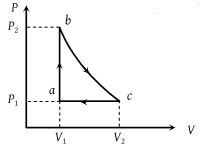(1) 4200 J

(2) 5000 J

(3) 9000 J

(4) 9800 J

Concept Questions :-

Cyclic process
High Yielding Test Series + Question Bank - NEET 2020

Difficulty Level:

A sample of ideal monoatomic gas is taken round the cycle CBAC as shown in the figure. The work done during the cycle is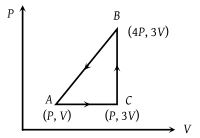(1) Zero

(2) 3 PV

(3) 6 PV

(4) 9PV

Concept Questions :-

Work done by gas
High Yielding Test Series + Question Bank - NEET 2020

Difficulty Level:

When a system is taken from state i to a state f along path iaf, Q = 50 J and W = 20 J. Along path ibf, Q = 35 J. If W = –13 J for the curved return path f i, Q for this path is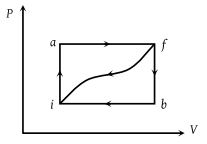(1) 33 J

(2) 23 J

(3) – 7 J

(4) – 43 J

Concept Questions :-

First law of thermodynamics
High Yielding Test Series + Question Bank - NEET 2020

Difficulty Level:

For one complete cycle of a thermodynamic process on a gas as shown in the P-V diagram, which of following is correct ?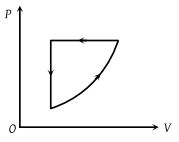(1) $\Delta {E}_{\mathrm{int}}=0,\text{\hspace{0.17em}}Q<0$

(2) $\Delta {E}_{\mathrm{int}}=0,\text{\hspace{0.17em}}Q>0$

(3) $\Delta \text{\hspace{0.17em}}{E}_{\mathrm{int}}>0,\text{\hspace{0.17em}}Q<0$

(4) $\Delta \text{\hspace{0.17em}}{E}_{\mathrm{int}}<0,\text{\hspace{0.17em}}Q>\text{​\hspace{0.17em}}0$

Concept Questions :-

Cyclic process
High Yielding Test Series + Question Bank - NEET 2020

Difficulty Level:

An ideal gas is taken from point A to the point B, as shown in the P-V diagram. The work done in the process is -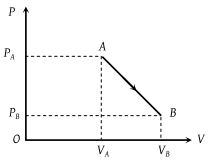(1) $\left({P}_{A}-{P}_{B}\right)\left({V}_{B}-{V}_{A}\right)$

(2) $\frac{1}{2}\left({P}_{B}-{P}_{A}\right)\left({V}_{B}+{V}_{A}\right)$

(3) $\frac{1}{2}\left({P}_{B}-{P}_{A}\right)\left({V}_{B}-{V}_{A}\right)$

(4) $\frac{1}{2}\left({P}_{B}+{P}_{A}\right)\left({V}_{B}-{V}_{A}\right)$

Concept Questions :-

Work done by gas
High Yielding Test Series + Question Bank - NEET 2020

Difficulty Level:

The P-V diagram of a system undergoing thermodynamic transformation is shown in figure. The work done by the system in going from AB C is 30J and 40J heat is given to the system. The change in internal energy between A and C is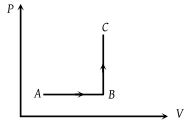(1) 10 J

(2) 70 J

(3) 84 J

(4) 134 J

Concept Questions :-

First law of thermodynamics
High Yielding Test Series + Question Bank - NEET 2020

Difficulty Level:

Consider a process shown in the figure. During this process the work done by the system -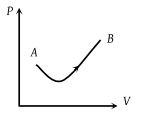(1) Continuously increases

(2) Continuously decreases

(3) First increases, then decreases

(4) First decreases, then increases

Concept Questions :-

Work done by gas
High Yielding Test Series + Question Bank - NEET 2020

Difficulty Level:

Six moles of an ideal gas perform a cycle shown in figure. If the temperature are TA = 600 K, TB = 800 K, TC = 2200 K and TD = 1200 K, the work done per cycle is -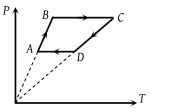(1) 20 kJ

(2) 30 kJ

(3) 40 kJ

(4) 60 kJ

Concept Questions :-

Types of processes
High Yielding Test Series + Question Bank - NEET 2020

Difficulty Level:

Which of the accompanying PV diagrams best represents an isothermal process ?

(1)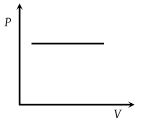(2)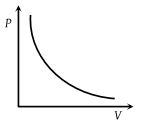(3)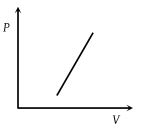(4)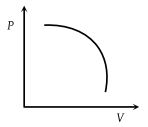Concept Questions :-

Types of processes
High Yielding Test Series + Question Bank - NEET 2020

Difficulty Level:

In the following figure, four curves A, B, C and D are shown. The curves are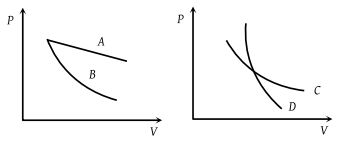(1) Isothermal for A and D while adiabatic for B and C

(2) Adiabatic for A and C while isothermal for B and D

(3) Isothermal for A and B while adiabatic for C and D

(4) Isothermal for A and C while adiabatic for B and D

Concept Questions :-

Types of processes